# NCERT Exemplar Class 10 Maths Solutions for Chapter 12 - Surface Areas And Volumes

## NCERT Exemplar Solutions Class 10 Maths Chapter 12 – Free PDF Download

NCERT Exemplar Solutions for Class 10 Maths Chapter 12 Surface Areas and Volumes are provided here in PDF so that students can download them and prepare well for the CBSE Board exams. These exemplar problems and solutions are designed by Maths experts with respect to the latest syllabus of CBSE (2023-2024).

Students can download the PDF of these solved questions for free and use them to get a clear idea about the important formulas as well as practise sums of surface areas and volumes to develop better Math skills. They can also  use this NCERT Exemplar to solve even the difficult questions and be ready for the board exams. Moreover, the sample papers and previous years’ question papers are of great help in getting familiar with the types of questions asked in the exam from Chapter 12 and the marking scheme. This chapter covers the following topics of Surface Areas and Volumes:

• Surface area of a combination of solid objects
• The volume of a combination of solid objects
• Frustum of a cone and finding the volume, the curved surface area, and the total surface area

In Chapter 12, students will learn about finding the surface areas and volumes of different types of solids, including cylinder, sphere, cuboid, or cone-shaped solids, as well as other combinations of solids. Dealing with this chapter can take some amount of time, and students will need to pay close attention. To help students easily solve chapter problems and find the right answers to difficult questions, the NCERT Exemplar for Class 10 Maths is provided here.

Students of Class 10 are also provided with online learning materials such as notes, exemplar books, NCERT solutions, and question papers to help them prepare well for the board exams.

## Download the PDF of NCERT Exemplar Class 10 Maths Solutions for Chapter 12 Surface Area and Volumes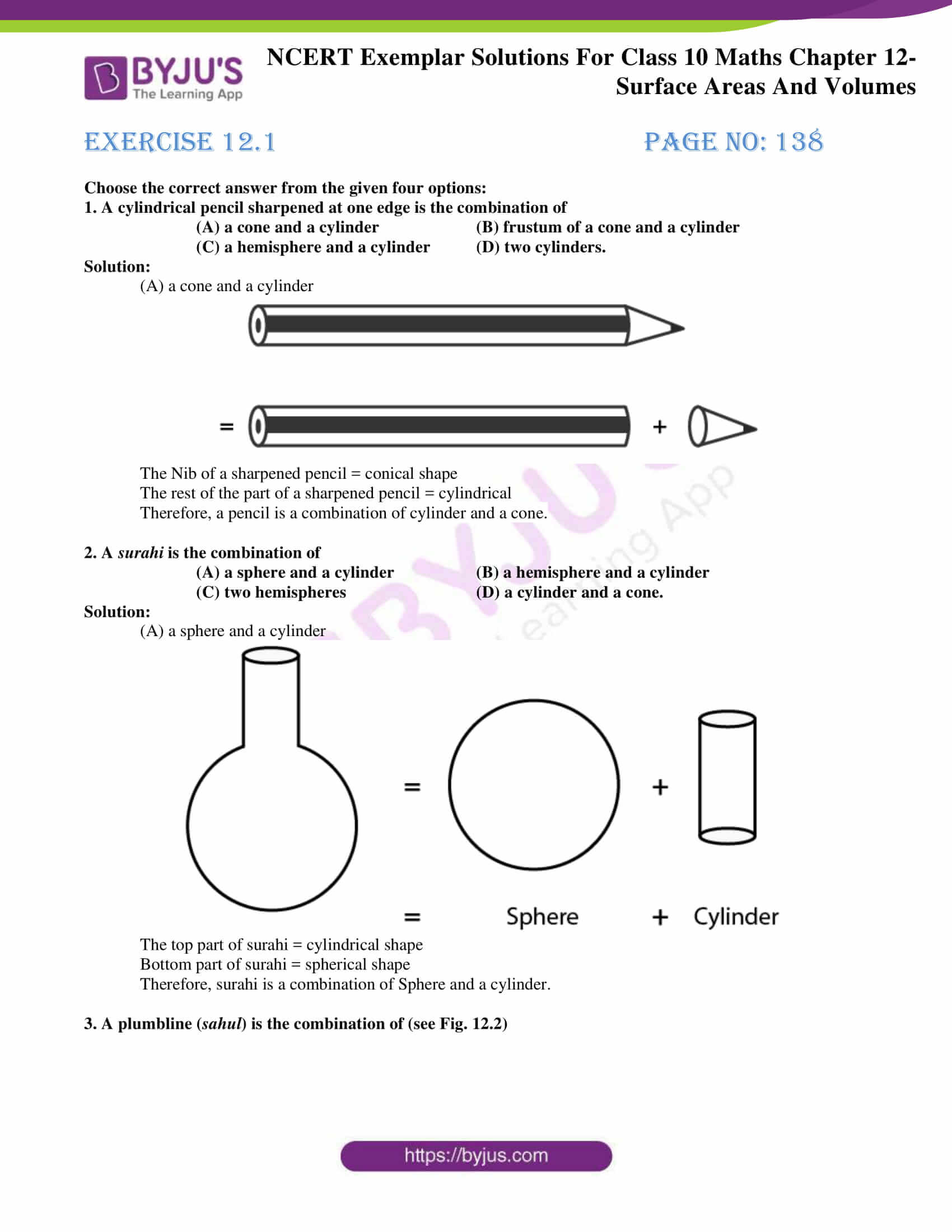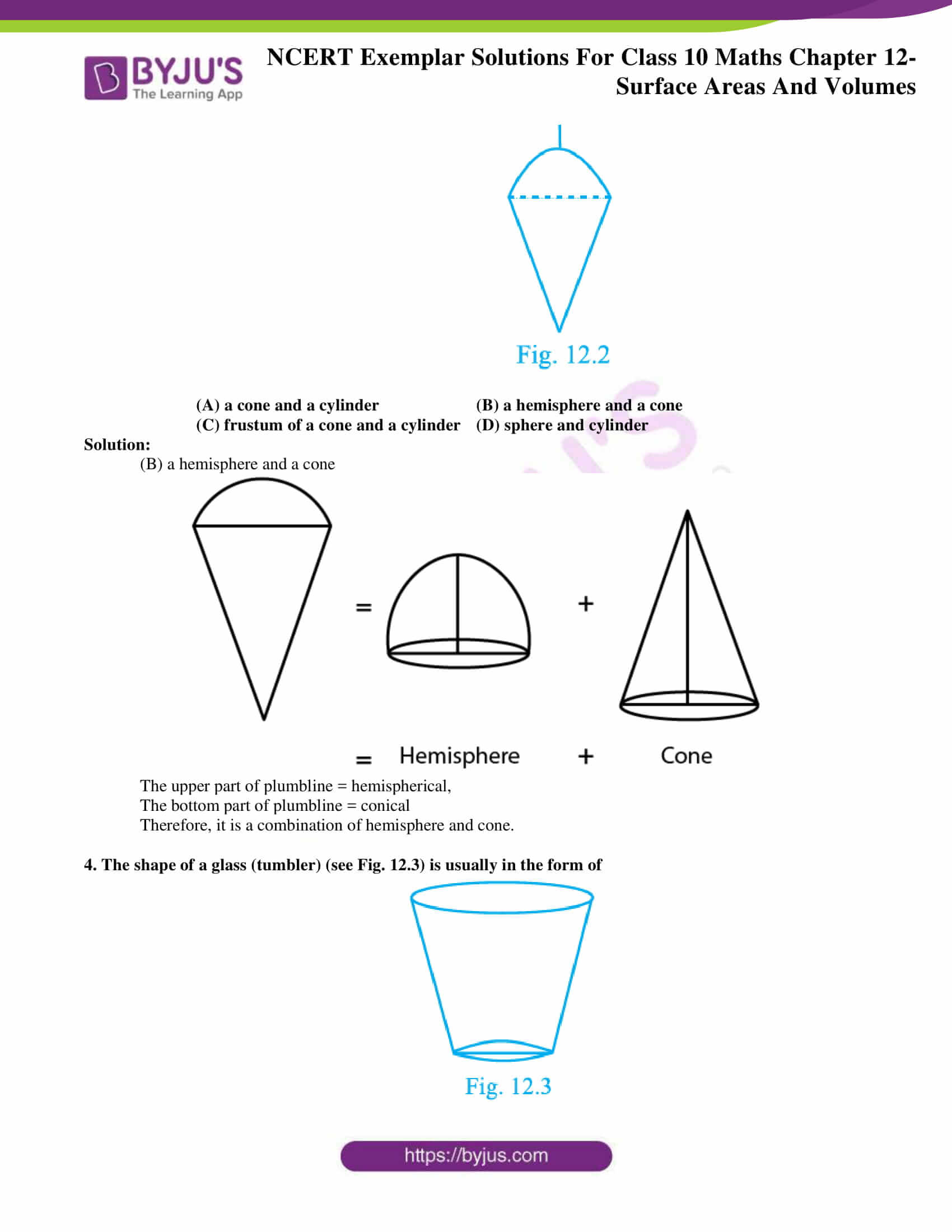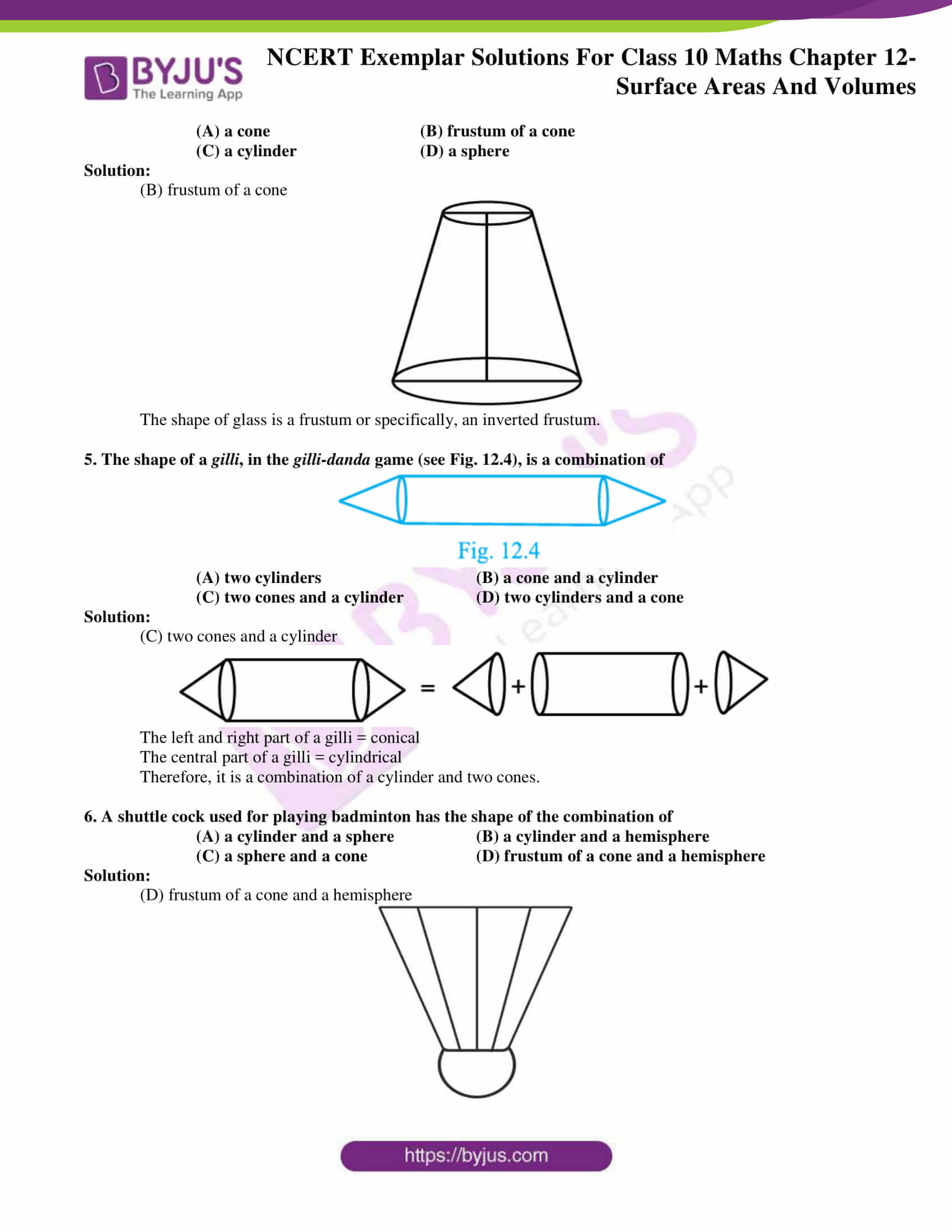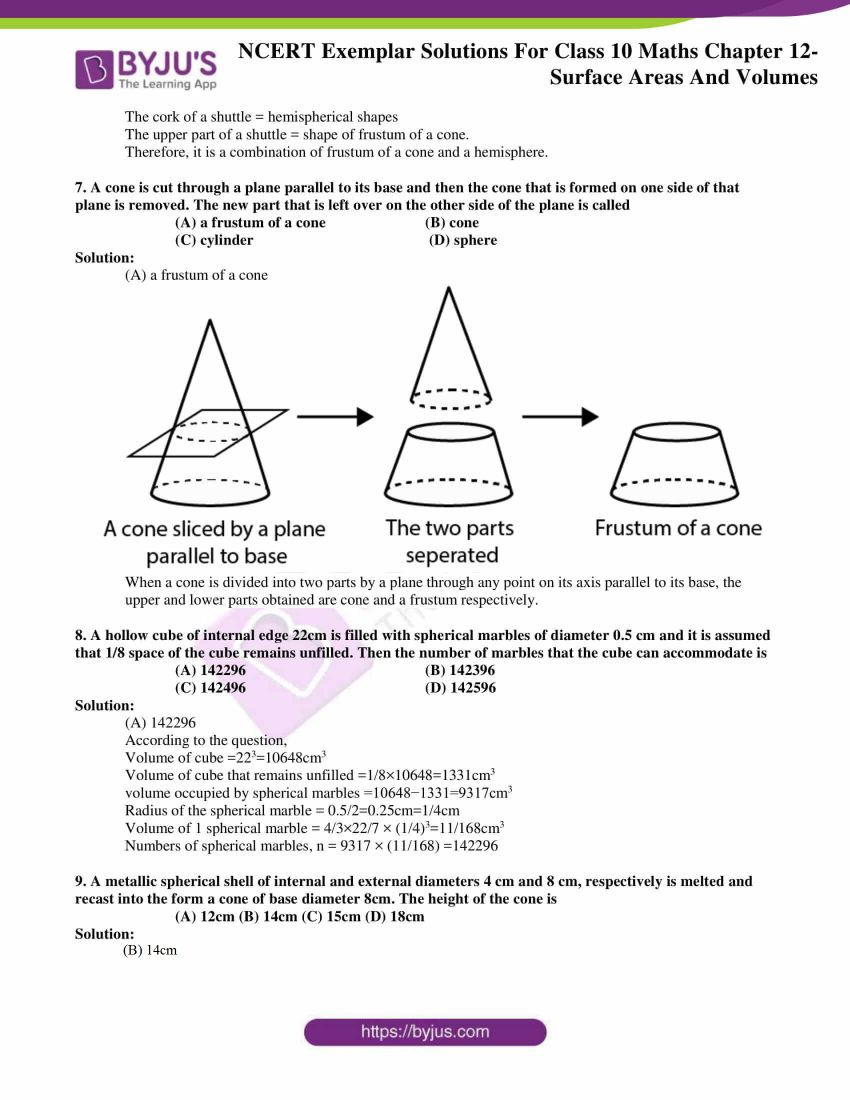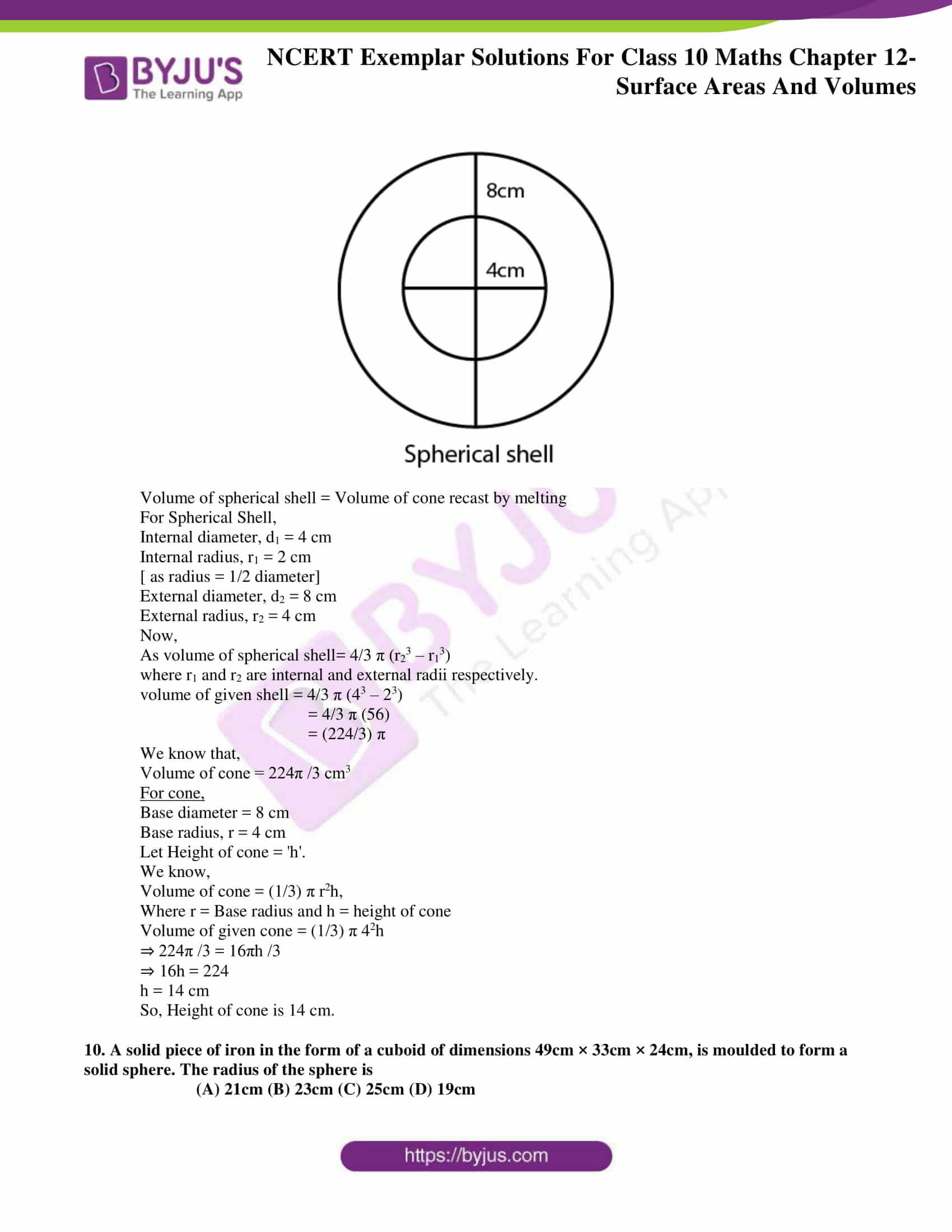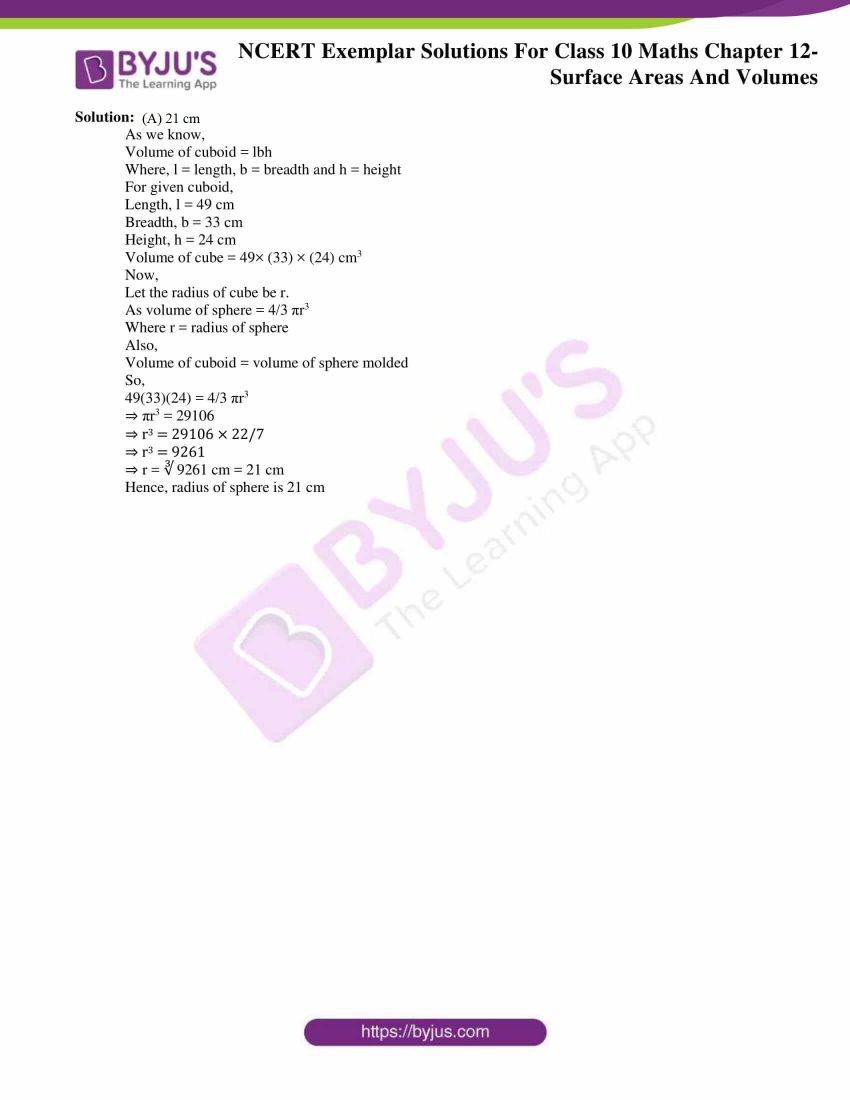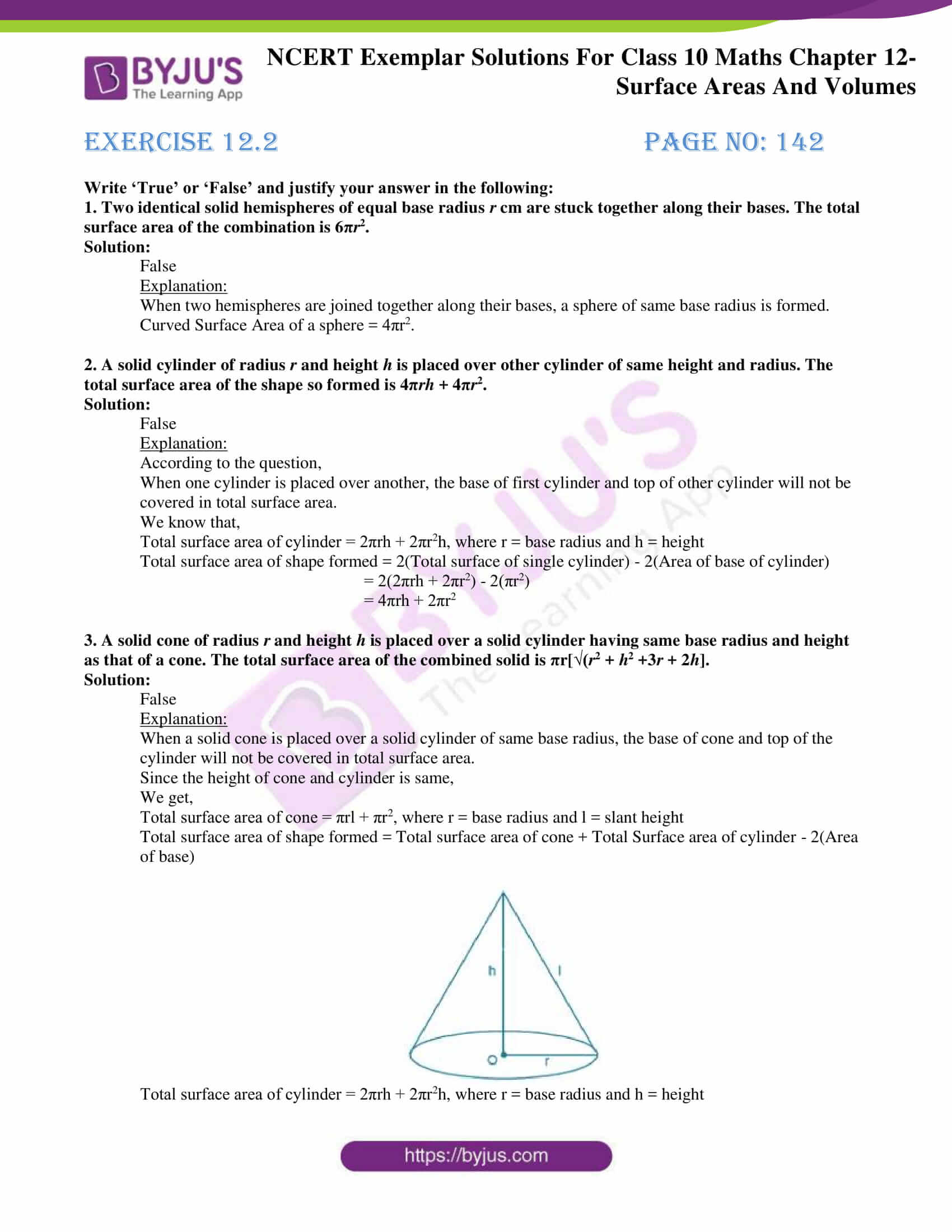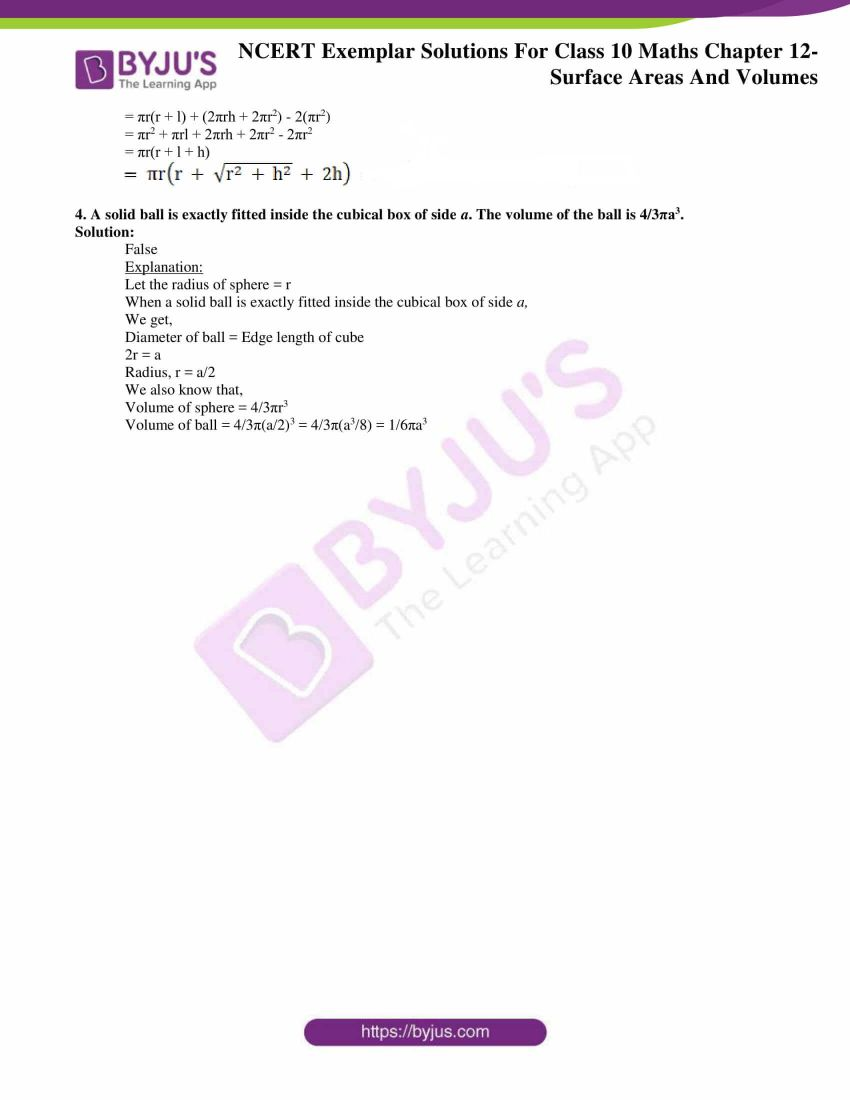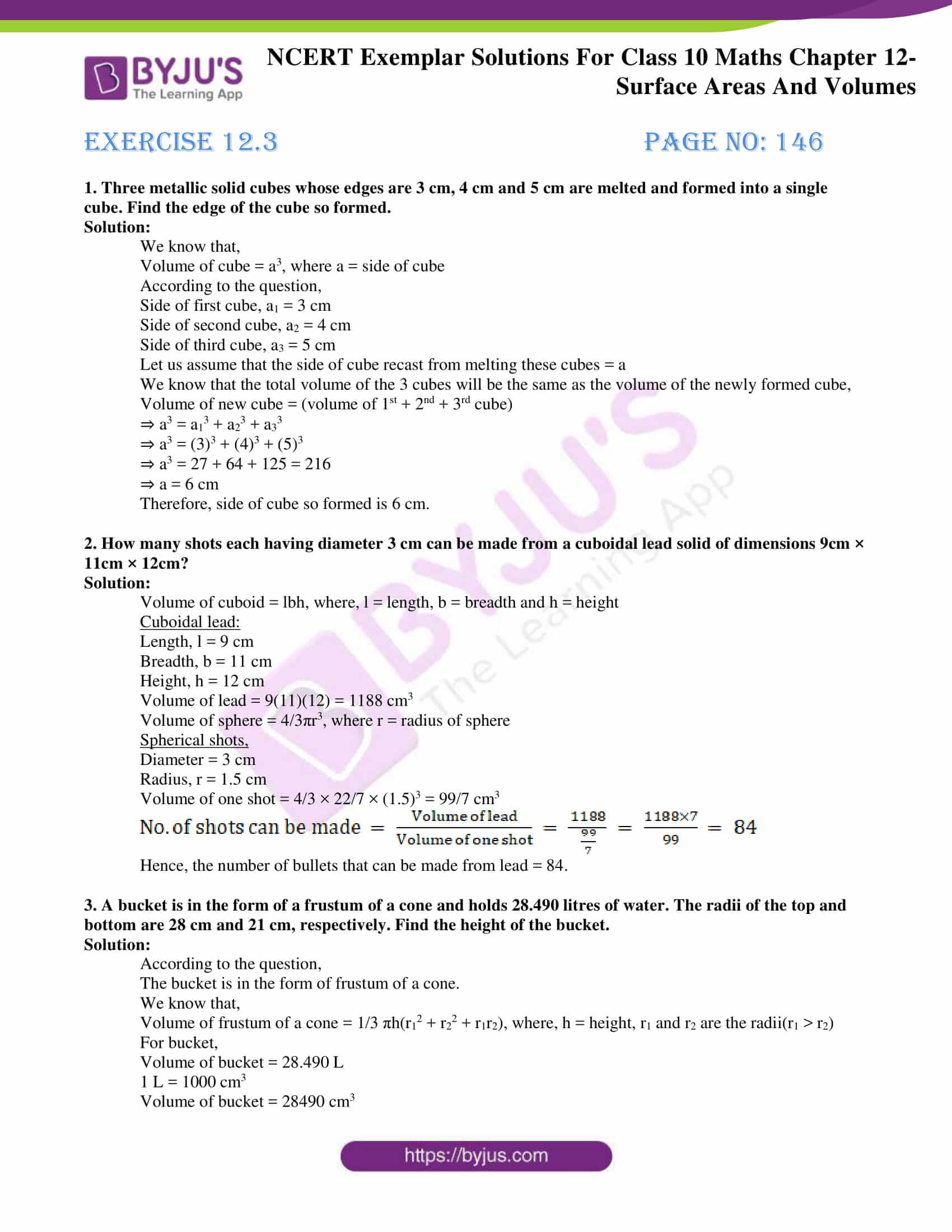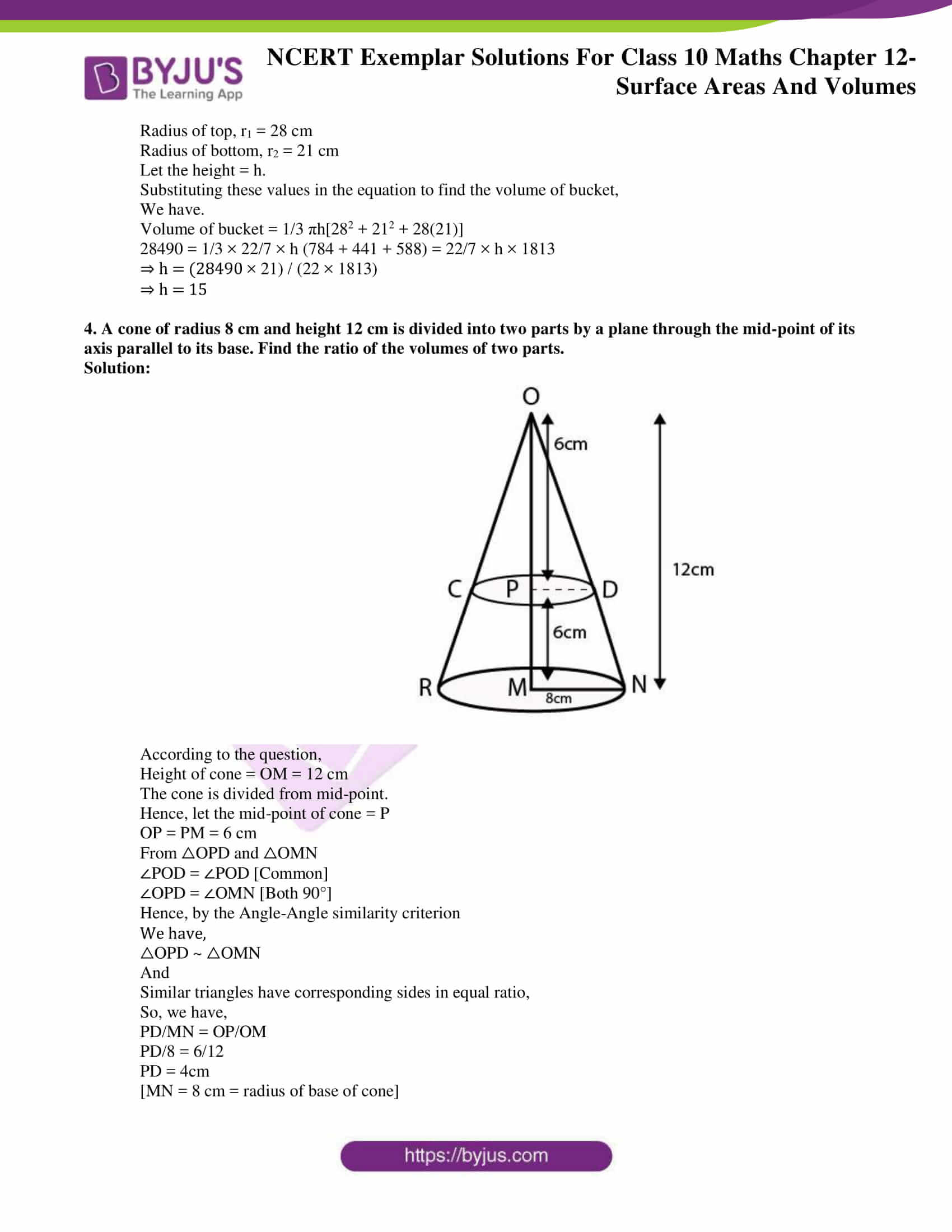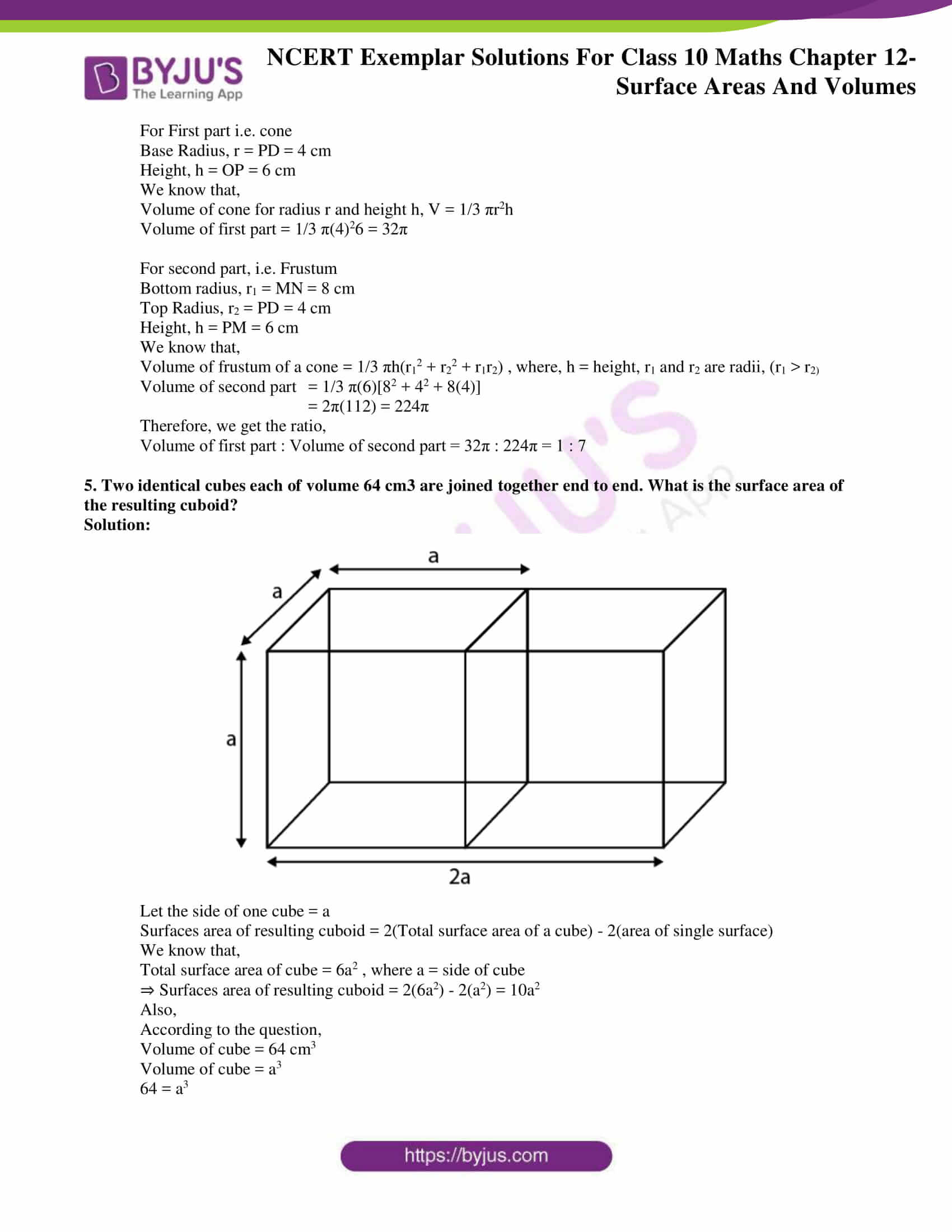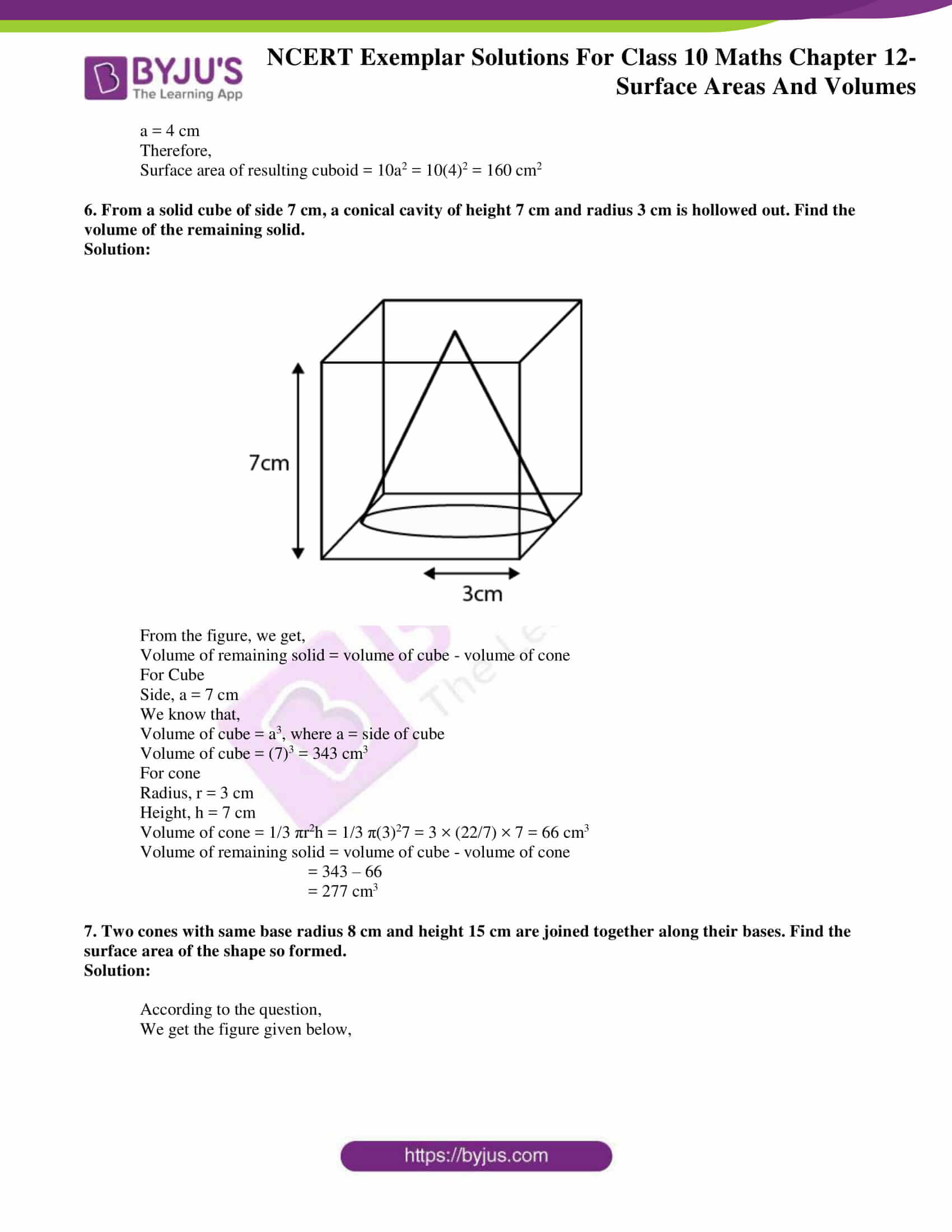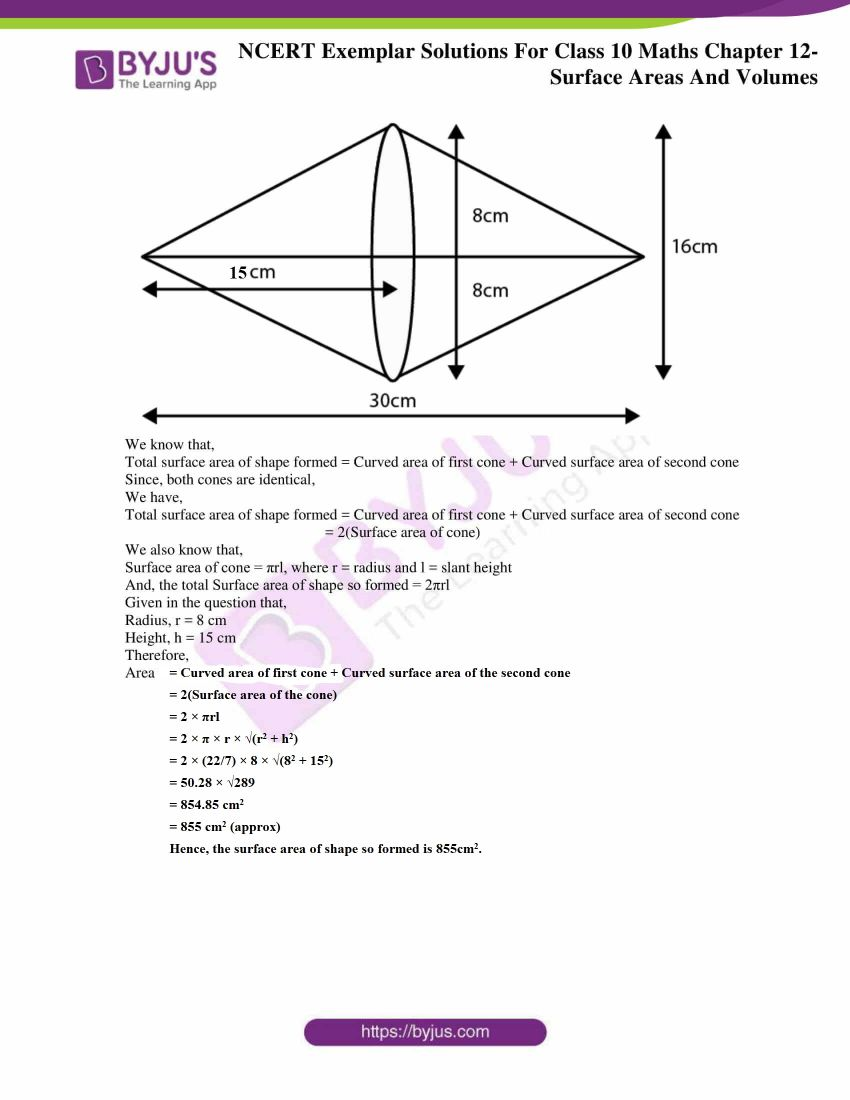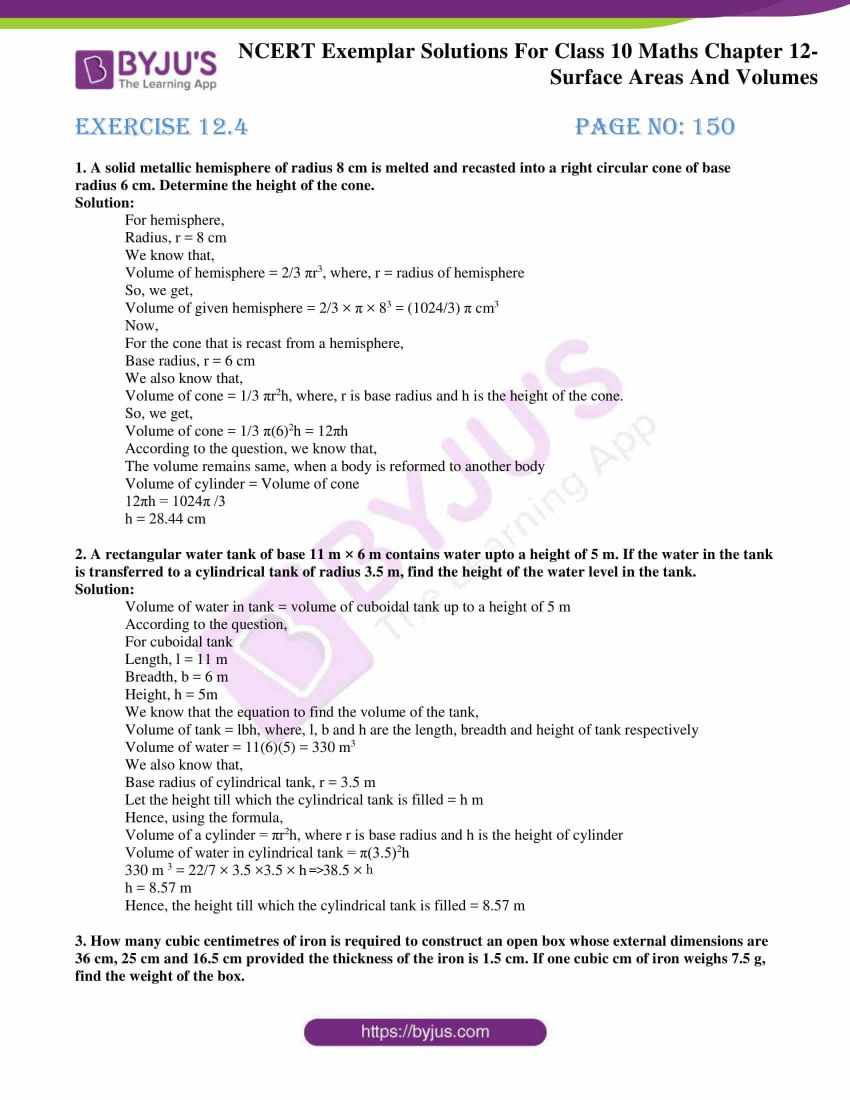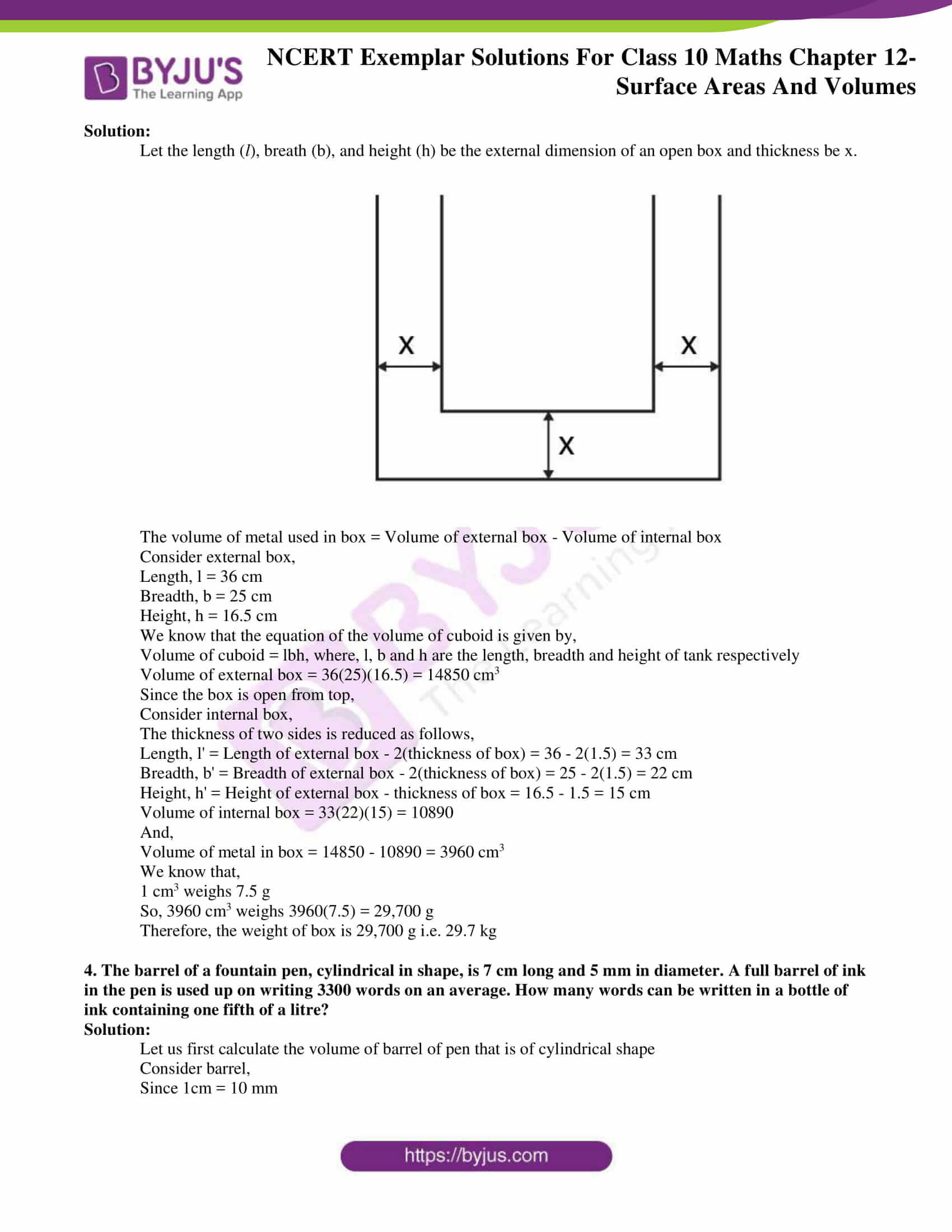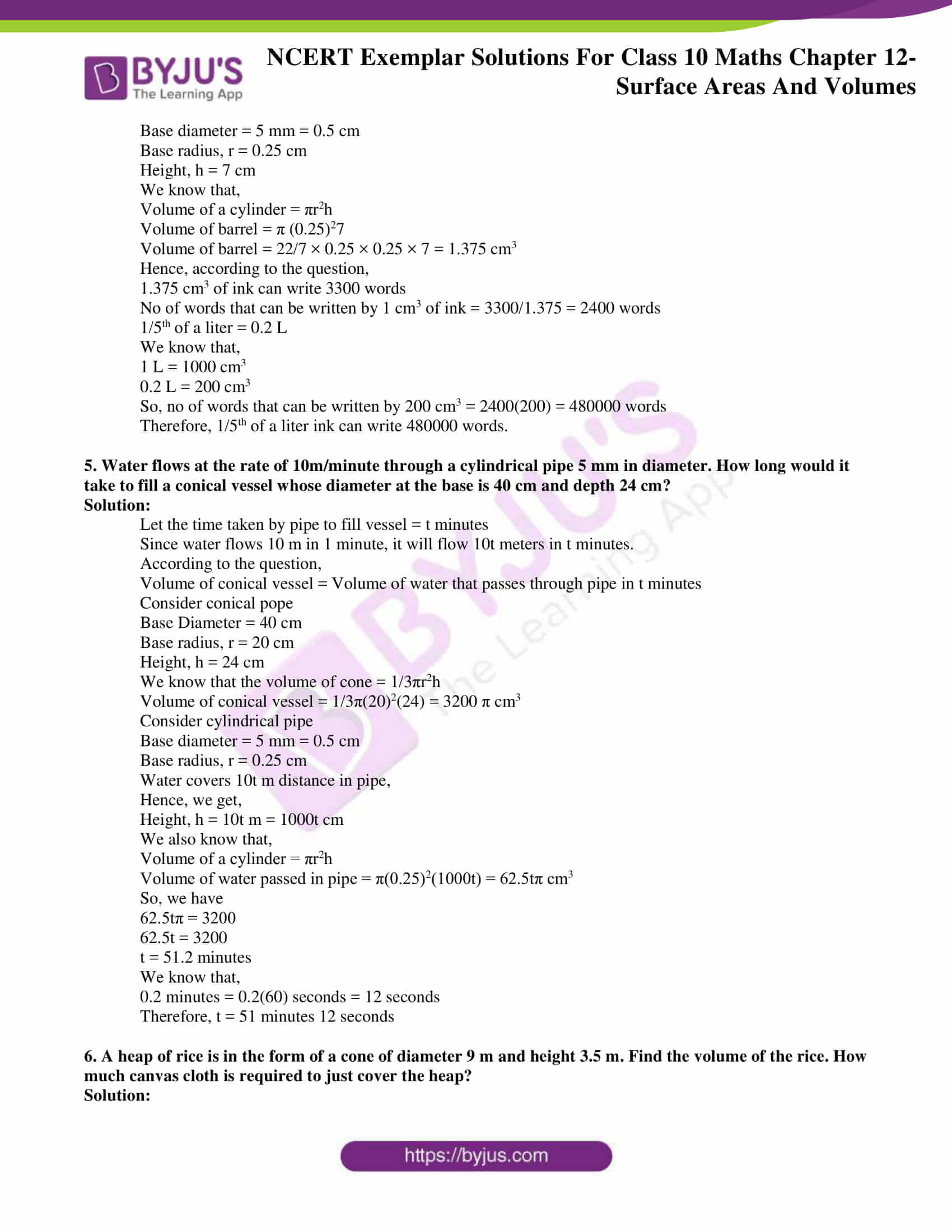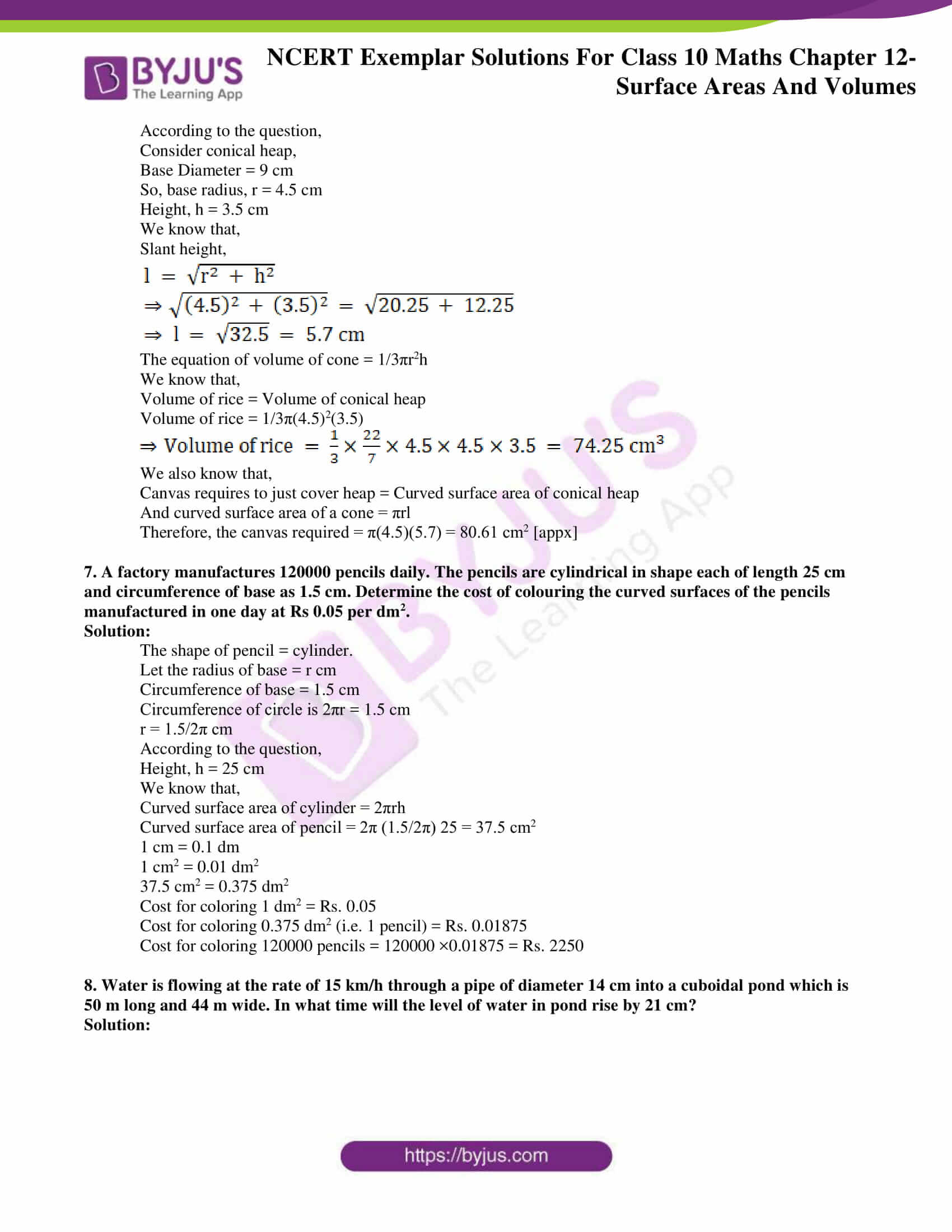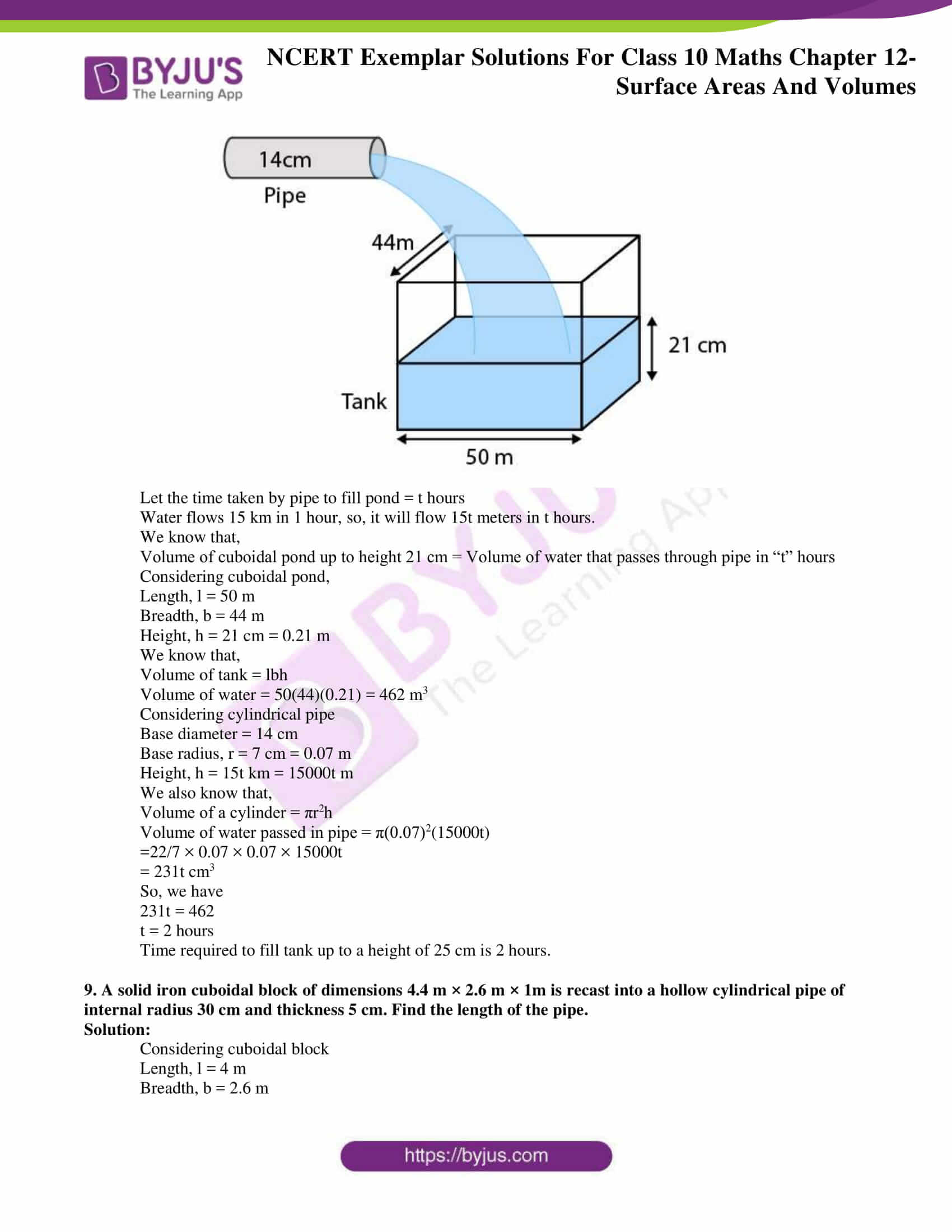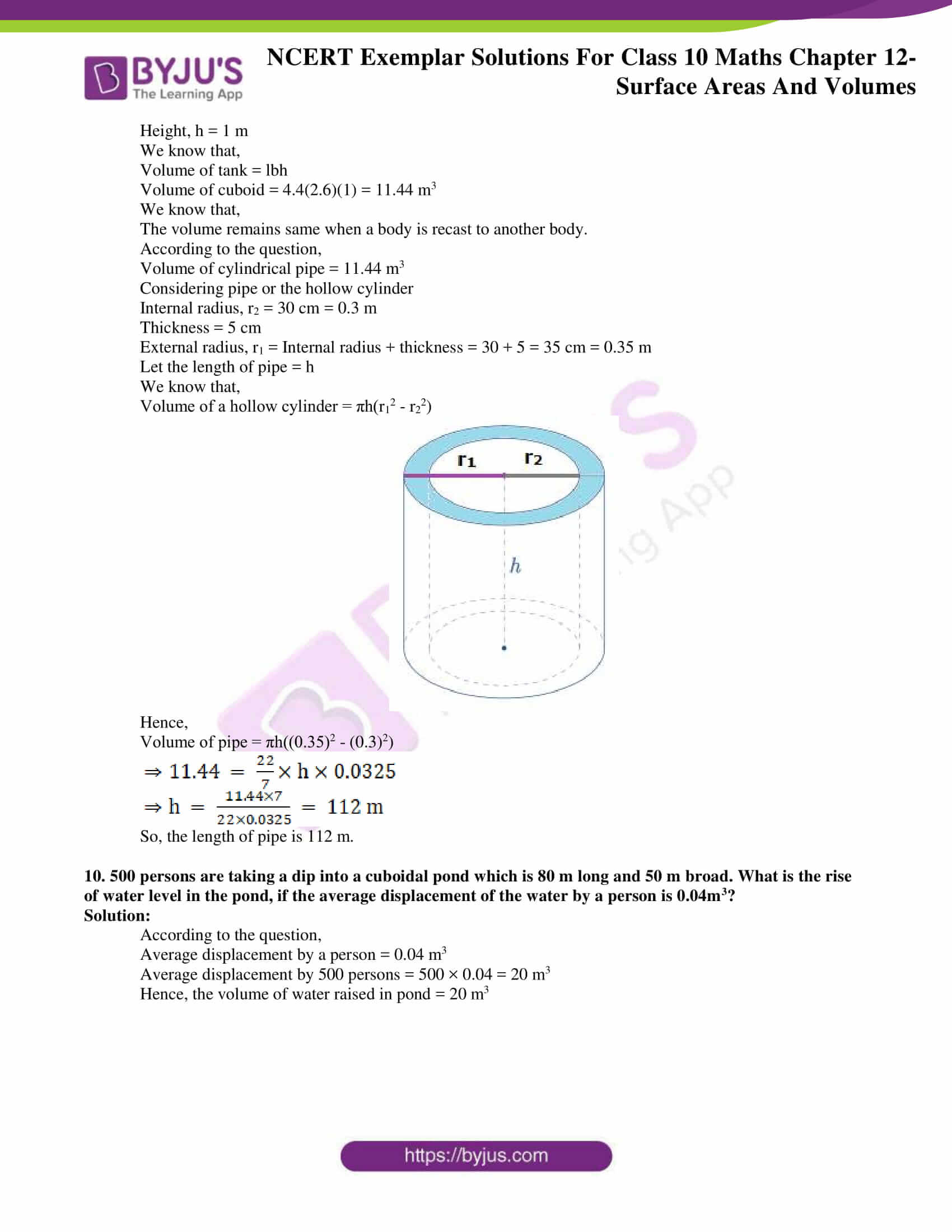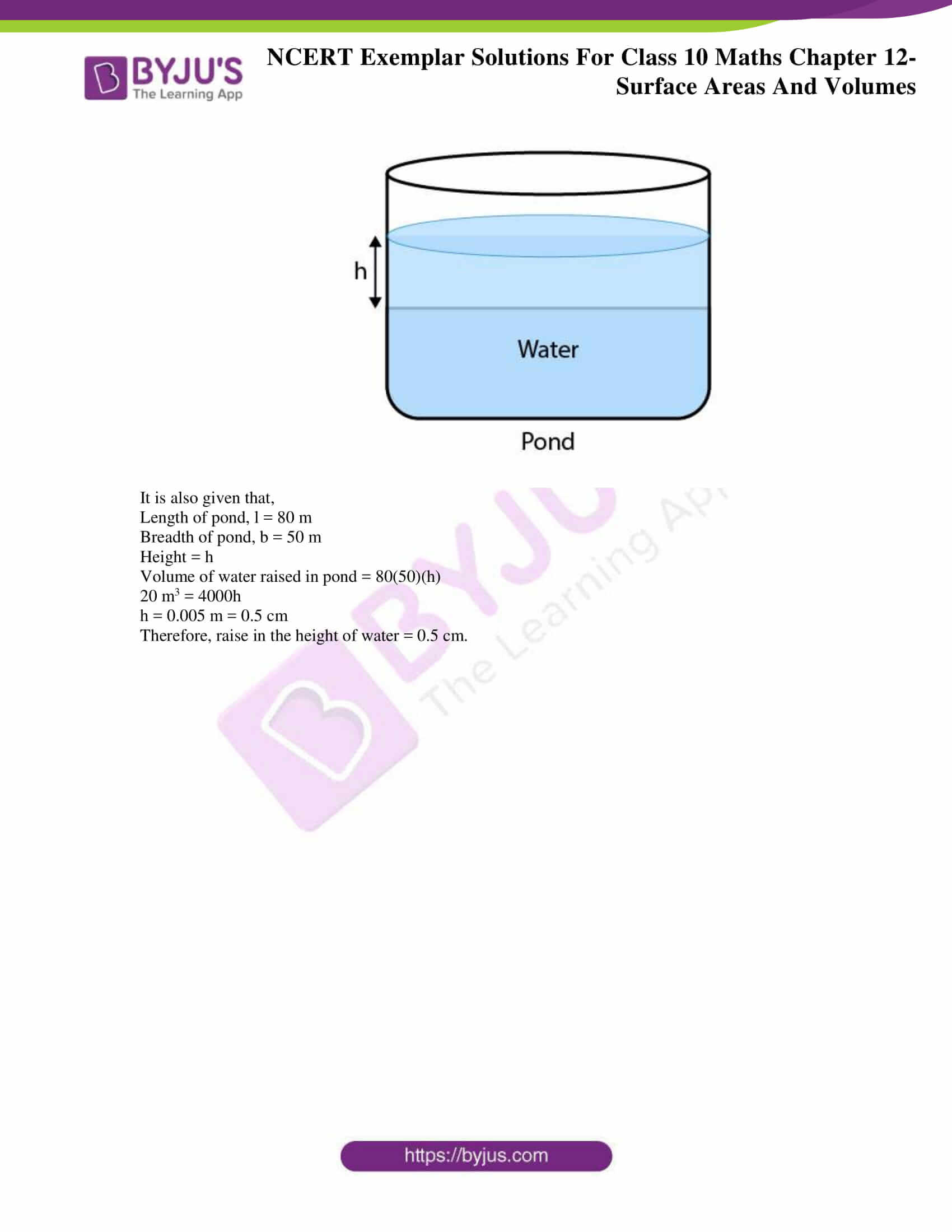## Exercise 12.1 Page No: 138

Choose the correct answer from the given four options:

1. A cylindrical pencil sharpened at one edge is the combination of

(A) a cone and a cylinder (B) frustum of a cone and a cylinder

(C) a hemisphere and a cylinder (D) two cylinders.

Solution:

(A) a cone and a cylinder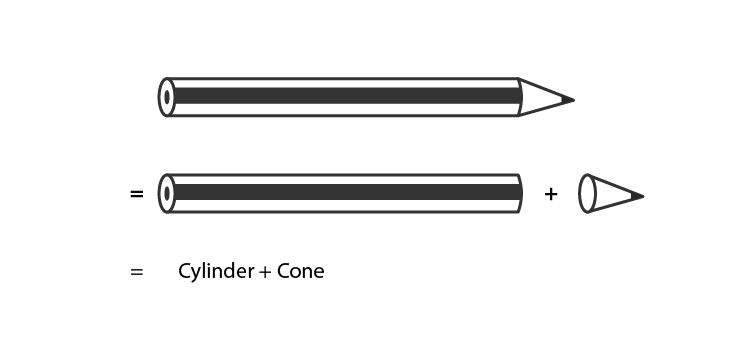The Nib of a sharpened pencil = conical shape

The rest of the part of a sharpened pencil = cylindrical

Therefore, a pencil is a combination of cylinder and a cone.

2. A surahi is the combination of

(A) a sphere and a cylinder (B) a hemisphere and a cylinder

(C) two hemispheres (D) a cylinder and a cone.

Solution:

(A) a sphere and a cylinder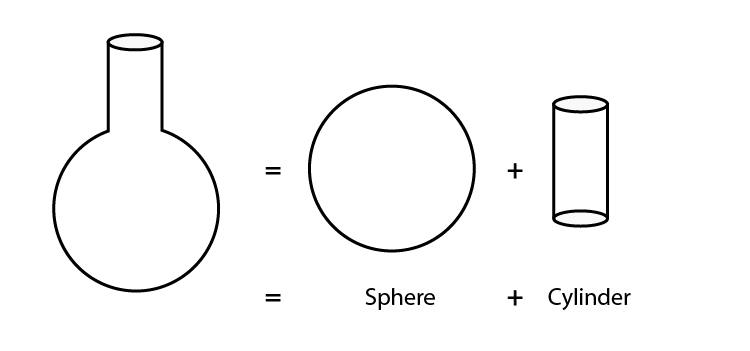The top part of surahi = cylindrical shape

Bottom part of surahi = spherical shape

Therefore, surahi is a combination of Sphere and a cylinder.

3. A plumbline (sahul) is the combination of (see Fig. 12.2)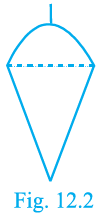(A) a cone and a cylinder (B) a hemisphere and a cone

(C) frustum of a cone and a cylinder (D) sphere and cylinder

Solution:

(B) a hemisphere and a cone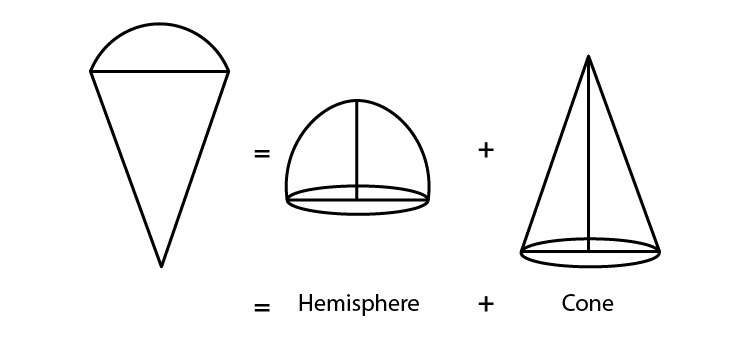The upper part of plumbline = hemispherical,

The bottom part of plumbline = conical

Therefore, it is a combination of hemisphere and cone.

4. The shape of a glass (tumbler) (see Fig. 12.3) is usually in the form of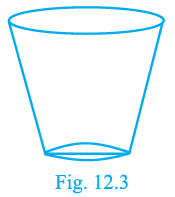(A) a cone (B) frustum of a cone

(C) a cylinder (D) a sphere

Solution:

(B) frustum of a cone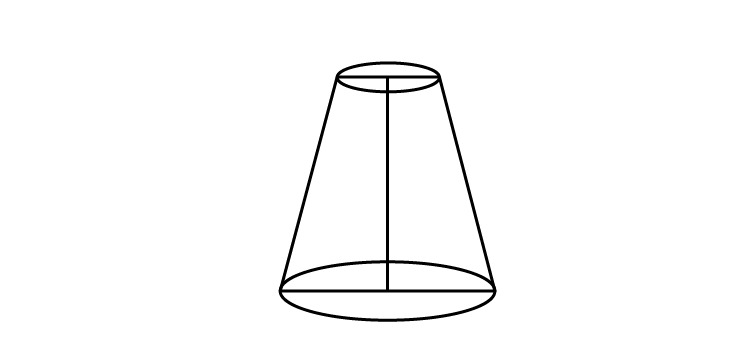The shape of glass is a frustum or specifically, an inverted frustum.

5. The shape of a gilli, in the gilli-danda game (see Fig. 12.4), is a combination of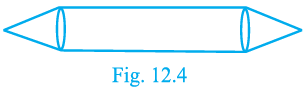(A) two cylinders (B) a cone and a cylinder

(C) two cones and a cylinder (D) two cylinders and a cone

Solution:

(C) two cones and a cylinder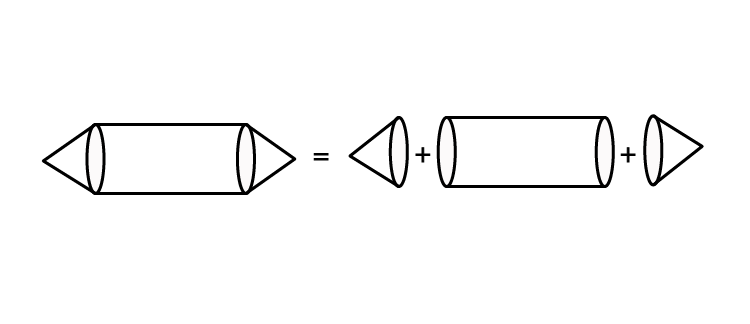The left and right part of a gilli = conical

The central part of a gilli = cylindrical

Therefore, it is a combination of a cylinder and two cones.

6. A shuttle cock used for playing badminton has the shape of the combination of

(A) a cylinder and a sphere (B) a cylinder and a hemisphere

(C) a sphere and a cone (D) frustum of a cone and a hemisphere

Solution:

(D) frustum of a cone and a hemisphere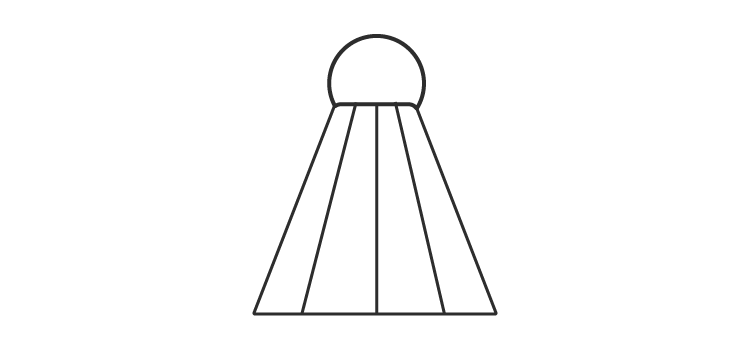The cork of a shuttle = hemispherical shapes

The upper part of a shuttle = shape of frustum of a cone.

Therefore, it is a combination of frustum of a cone and a hemisphere.

7. A cone is cut through a plane parallel to its base and then the cone that is formed on one side of that plane is removed. The new part that is left over on the other side of the plane is called

(A) a frustum of a cone (B) cone

(C) cylinder (D) sphere

Solution:

(A) a frustum of a cone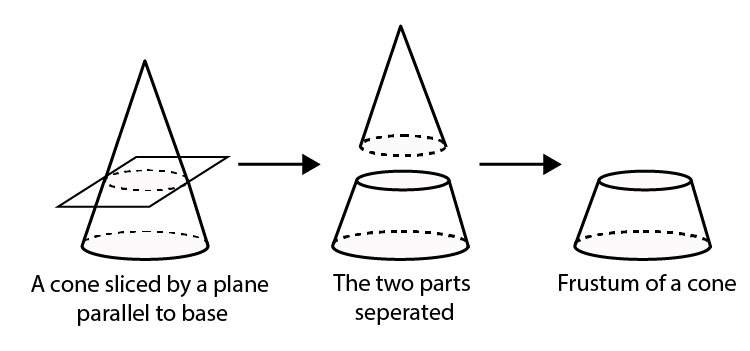When a cone is divided into two parts by a plane through any point on its axis parallel to its base, the upper and lower parts obtained are cone and a frustum, respectively.

8. A hollow cube of internal edge 22cm is filled with spherical marbles of diameter 0.5 cm and it is assumed that 1/8 space of the cube remains unfilled. Then the number of marbles that the cube can accommodate is

(A) 142296 (B) 142396

(C) 142496 (D) 142596

Solution:

(A) 142296

According to the question,

Volume of cube =223=10648cm3

Volume of cube that remains unfilled =1/8×10648=1331cm3

volume occupied by spherical marbles =10648−1331=9317cm3

Radius of the spherical marble = 0.5/2=0.25cm=1/4cm

Volume of 1 spherical marble = 4/3×22/7 × (1/4)3=11/168cm3

Numbers of spherical marbles, n = 9317 × (11/168) =142296

9. A metallic spherical shell of internal and external diameters 4 cm and 8 cm, respectively is melted and recast into the form a cone of base diameter 8cm. The height of the cone is

(A) 12cm (B) 14cm (C) 15cm (D) 18cm

Solution:

(B) 14cm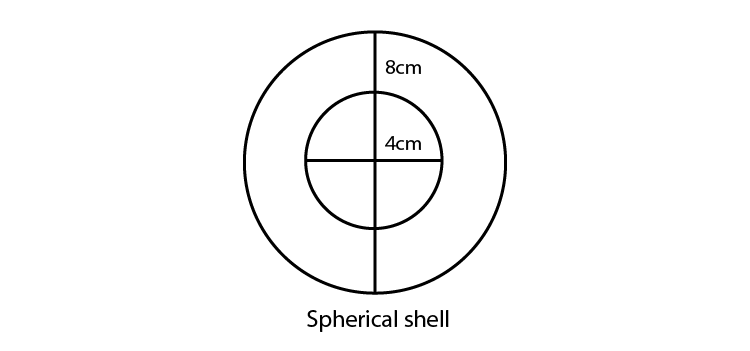Volume of spherical shell = Volume of cone recast by melting

For Spherical Shell,

Internal diameter, d1 = 4 cm

Internal radius, r1 = 2 cm

[ as radius = 1/2 diameter]

External diameter, d2 = 8 cm

External radius, r2 = 4 cm

Now,

As volume of spherical shell= 4/3 π (r23 – r13)

where r1 and r2 are internal and external radii respectively.

volume of given shell = 4/3 π (43 – 23)

= 4/3 π (56)

= (224/3) π

We know that,

Volume of cone = 224π /3 cm3

For cone,

Base diameter = 8 cm

Base radius, r = 4 cm

Let Height of cone = ‘h’.

We know,

Volume of cone = (1/3) π r2h,

Where r = Base radius and h = height of cone

Volume of given cone = (1/3) π 42h

⇒ 224π /3 = 16πh /3

⇒ 16h = 224

h = 14 cm

So, Height of cone is 14 cm.

10. A solid piece of iron in the form of a cuboid of dimensions 49cm × 33cm × 24cm, is moulded to form a solid sphere. The radius of the sphere is

(A) 21cm (B) 23cm (C) 25cm (D) 19cm

Solution:

(A) 21cm

As we know,

Volume of cuboid = lbh

Where, l = length, b = breadth and h = height

For given cuboid,

Length, l = 49 cm

Height, h = 24 cm

Volume of cube = 49× (33) × (24) cm3

Now,

Let the radius of cube be r.

As volume of sphere = 4/3 πr3

Where r = radius of sphere

Also,

Volume of cuboid = volume of sphere moulded

So,

49(33)(24) = 4/3 πr3

⇒ πr3 = 29106

⇒ r3 = 29106 × 22/7

⇒ r3 = 9261

⇒ r = ∛ 9261 cm = 21 cm

Hence, radius of sphere is 21 cm

## Exercise 12.2 Page No: 142

1. Two identical solid hemispheres of equal base radius r cm are stuck together along their bases. The total surface area of the combination is 6πr2.

Solution:

False

Explanation:

When two hemispheres are joined together along their bases, a sphere of same base radius is formed.

Curved Surface Area of a sphere = 4πr2.

2. A solid cylinder of radius r and height h is placed over another cylinder of the same height and radius. The total surface area of the shape so formed is 4πrh + 4πr2.

Solution:

False

Explanation:

According to the question,

When one cylinder is placed over another, the base of first cylinder and top of another cylinder will not be covered in total surface area.

We know that,

Total surface area of cylinder = 2πrh + 2πr2h, where r = base radius and h = height

Total surface area of shape formed = 2(Total surface of single cylinder) – 2(Area of base of cylinder)

= 2(2πrh + 2πr2) – 2(πr2)

= 4πrh + 2πr2

3. A solid cone of radius r and height h is placed over a solid cylinder having same base radius and height as that of a cone. The total surface area of the combined solid is πr[√(r2 + h2 +3r + 2h].

Solution:

False

Explanation:

When a solid cone is placed over a solid cylinder of same base radius, the base of cone and top of the cylinder will not be covered in total surface area.

Since the height of cone and cylinder is same,

We get,

Total surface area of cone = πrl + πr2, where r = base radius and l = slant height

Total surface area of shape formed = Total surface area of cone + Total Surface area of cylinder – 2(Area of base)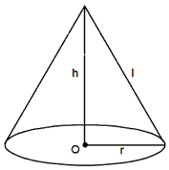Total surface area of cylinder = 2πrh + 2πr2h, where r = base radius and h = height

= πr(r + l) + (2πrh + 2πr2) – 2(πr2)

= πr2 + πrl + 2πrh + 2πr2 – 2πr2

= πr(r + l + h)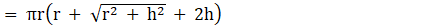4. A solid ball is exactly fitted inside the cubical box of side a. The volume of the ball is 4/3πa3.

Solution:

False

Explanation:

Let the radius of sphere = r

When a solid ball is exactly fitted inside the cubical box of side a,

We get,

Diameter of ball = Edge length of cube

2r = a

We also know that,

Volume of sphere = 4/3πr3

Volume of ball = 4/3π(a/2)3 = 4/3π(a3/8) = 1/6πa3

## Exercise 12.3 Page No: 146

1. Three metallic solid cubes whose edges are 3 cm, 4 cm and 5 cm are melted and formed into a single cube. Find the edge of the cube so formed.

Solution:

We know that,

Volume of cube = a3, where a = side of cube

According to the question,

Side of first cube, a1 = 3 cm

Side of second cube, a2 = 4 cm

Side of third cube, a3 = 5 cm

Let us assume that the side of cube recast from melting these cubes = a

We know that the total volume of the 3 cubes will be the same as the volume of the newly formed cube,

Volume of new cube = (volume of 1st + 2nd + 3rd cube)

⇒ a3 = a13 + a23 + a33

⇒ a3 = (3)3 + (4)3 + (5)3

⇒ a3 = 27 + 64 + 125 = 216

⇒ a = 6 cm

Therefore, side of cube so formed is 6 cm.

2. How many shots each having diameter 3 cm can be made from a cuboidal lead solid of dimensions 9cm × 11cm × 12cm?

Solution:

Volume of cuboid = lbh, where, l = length, b = breadth and h = height

Length, l = 9 cm

Height, h = 12 cm

Volume of lead = 9(11)(12) = 1188 cm3

Volume of sphere = 4/3πr3, where r = radius of sphere

Spherical shots,

Diameter = 3 cm

Volume of one shot = 4/3 × 22/7 × (1.5)3 = 99/7 cm3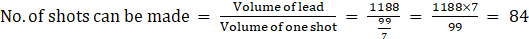Hence, the number of bullets that can be made from lead = 84.

3. A bucket is in the form of a frustum of a cone and holds 28.490 litres of water. The radii of the top and bottom are 28 cm and 21 cm, respectively. Find the height of the bucket.

Solution:

According to the question,

The bucket is in the form of frustum of a cone.

We know that,

Volume of frustum of a cone = 1/3 πh(r12 + r22 + r1r2), where, h = height, r1 and r2 are the radii(r1 > r2)

For bucket,

Volume of bucket = 28.490 L

1 L = 1000 cm3

Volume of bucket = 28490 cm3

Radius of top, r1 = 28 cm

Radius of bottom, r2 = 21 cm

Let the height = h.

Substituting these values in the equation to find the volume of bucket,

We have.

Volume of bucket = 1/3 πh[282 + 212 + 28(21)]

28490 = 1/3 × 22/7 × h (784 + 441 + 588) = 22/7 × h × 1813

⇒ h = (28490 × 21) / (22 × 1813)

⇒ h = 15

4. A cone of radius 8 cm and height 12 cm is divided into two parts by a plane through the mid-point of its axis parallel to its base. Find the ratio of the volumes of two parts.

Solution: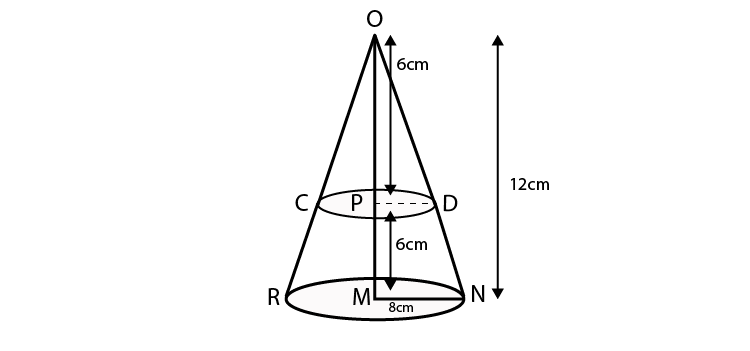According to the question,

Height of cone = OM = 12 cm

The cone is divided from mid-point.

Hence, let the mid-point of cone = P

OP = PM = 6 cm

From △OPD and △OMN

∠POD = ∠POD [Common]

∠OPD = ∠OMN [Both 90°]

Hence, by the Angle-Angle similarity criterion

We have,

△OPD ~ △OMN

And

Similar triangles have corresponding sides in equal ratio,

So, we have,

PD/MN = OP/OM

PD/8 = 6/12

PD = 4cm

[MN = 8 cm = radius of base of cone]

For First part i.e. cone

Base Radius, r = PD = 4 cm

Height, h = OP = 6 cm

We know that,

Volume of cone for radius r and height h, V = 1/3 πr2h

Volume of first part = 1/3 π(4)26 = 32π

For second part, i.e. Frustum

Bottom radius, r1 = MN = 8 cm

Top Radius, r2 = PD = 4 cm

Height, h = PM = 6 cm

We know that,

Volume of frustum of a cone = 1/3 πh(r12 + r22 + r1r2) , where, h = height, r1 and r2 are radii, (r1 > r2)

Volume of second part = 1/3 π(6)[82 + 42 + 8(4)]

= 2π(112) = 224π

Therefore, we get the ratio,

Volume of first part : Volume of second part = 32π : 224π = 1 : 7

5. Two identical cubes each of volume 64 cm3 are joined together end to end. What is the surface area of the resulting cuboid?

Solution: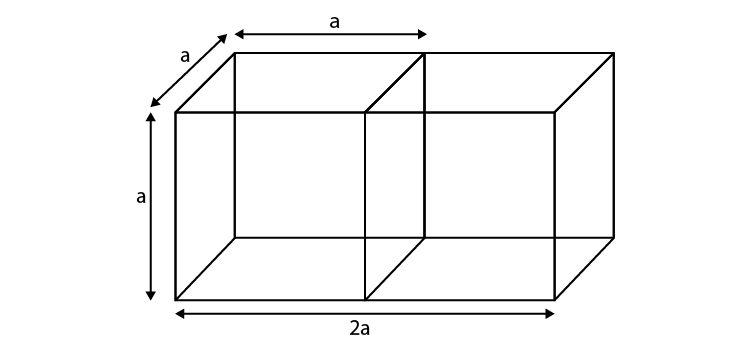Let the side of one cube = a

Surfaces area of resulting cuboid = 2(Total surface area of a cube) – 2(area of single surface)

We know that,

Total surface area of cube = 6a2 , where a = side of cube

⇒ Surfaces area of resulting cuboid = 2(6a2) – 2(a2) = 10a2

Also,

According to the question,

Volume of cube = 64 cm3

Volume of cube = a3

64 = a3

a = 4 cm

Therefore,

Surface area of resulting cuboid = 10a2 = 10(4)2 = 160 cm2

6. From a solid cube of side 7 cm, a conical cavity of height 7 cm and radius 3 cm is hollowed out. Find the volume of the remaining solid.

Solution: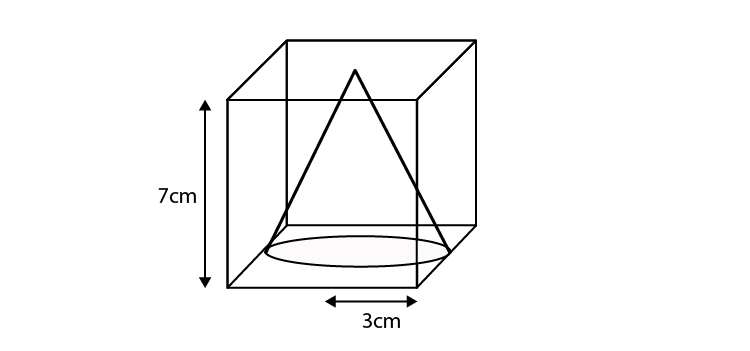From the figure, we get,

Volume of remaining solid = volume of cube – volume of cone

For Cube

Side, a = 7 cm

We know that,

Volume of cube = a3, where a = side of cube

Volume of cube = (7)3 = 343 cm3

For cone

Height, h = 7 cm

Volume of cone = 1/3 πr2h = 1/3 π(3)27 = 3 × (22/7) × 7 = 66 cm3

Volume of remaining solid = volume of cube – volume of cone

= 343 – 66

= 277 cm3

7. Two cones with same base radius 8 cm and height 15 cm are joined together along their bases. Find the surface area of the shape so formed.

Solution:

According to the question,

We get the figure given below,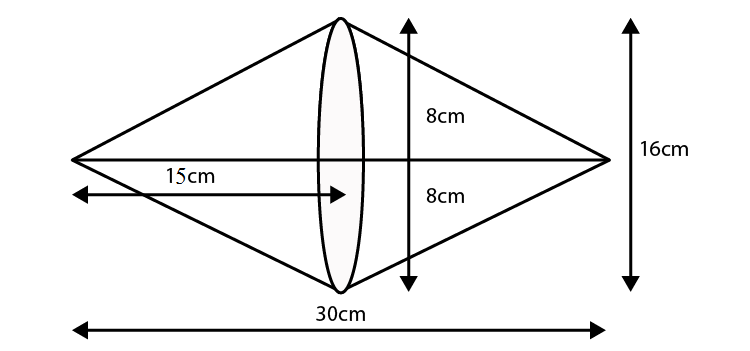We know that,

Total surface area of shape formed = Curved area of first cone + Curved surface area of second cone

Since, both cones are identical,

We have,

Total surface area of shape formed = Curved area of first cone + Curved surface area of the second cone

= 2(Surface area of cone)

We also know that,

Surface area of cone = πrl, where r = radius and l = slant height

And, the total Surface area of shape so formed = 2πrl

Given in the question that,

Height, h = 15 cm

Therefore,

Area = Curved area of first cone + Curved surface area of the second cone

= 2 (Surface area of the cone)

= 2 × πrl

= 2 × π × r × √(r2 + h2)

= 2 × (22/7) × 8 × √(82 + 152)

= 50.28 × √289

= 854.85 cm2

= 855 cm2 (approx)

Hence, the surface area of shape so formed is 855cm2.

## Exercise 12.4 Page No: 150

1. A solid metallic hemisphere of radius 8 cm is melted and recasted into a right circular cone of base radius 6 cm. Determine the height of the cone.

Solution:

For hemisphere,

We know that,

Volume of hemisphere = 2/3 πr3, where, r = radius of hemisphere

So, we get,

Volume of given hemisphere = 2/3 × π × 83 = (1024/3) π cm3

Now,

For the cone that is recast from a hemisphere,

Base radius, r = 6 cm

We also know that,

Volume of cone = 1/3 πr2h, where, r is base radius and h is the height of the cone.

So, we get,

Volume of cone = 1/3 π(6)2h = 12πh

According to the question, we know that,

The volume remains same, when a body is reformed to another body

Volume of cylinder = Volume of cone

12πh = 1024π /3

h = 28.44 cm

2. A rectangular water tank of base 11 m × 6 m contains water upto a height of 5 m. If the water in the tank is transferred to a cylindrical tank of radius 3.5 m, find the height of the water level in the tank.

Solution:

Volume of water in tank = volume of cuboidal tank up to a height of 5 m

According to the question,

For cuboidal tank

Length, l = 11 m

Height, h = 5m

We know that the equation to find the volume of the tank,

Volume of tank = lbh, where, l, b and h are the length, breadth and height of tank respectively

Volume of water = 11(6)(5) = 330 m3

We also know that,

Base radius of cylindrical tank, r = 3.5 m

Let the height till which the cylindrical tank is filled = h m

Hence, using the formula,

Volume of a cylinder = πr2h, where r is base radius and h is the height of cylinder

Volume of water in cylindrical tank = π(3.5)2h

330 m 3 = 22/7 × 3.5 ×3.5 × h

330 m 3 = h × 38.5

h = 8.57 m

Hence, the height till which the cylindrical tank is filled = 8.57 m

3. How many cubic centimetres of iron is required to construct an open box whose external dimensions are 36 cm, 25 cm and 16.5 cm provided the thickness of the iron is 1.5 cm. If one cubic cm of iron weighs 7.5 g, find the weight of the box.

Solution:

Let the length (l), breath (b), and height (h) be the external dimension of an open box and thickness be x.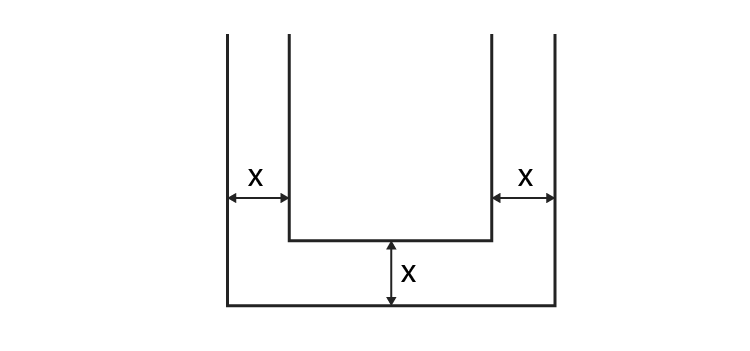The volume of metal used in box = Volume of external box – Volume of internal box

Consider external box,

Length, l = 36 cm

Height, h = 16.5 cm

We know that the equation of the volume of cuboid is given by,

Volume of cuboid = lbh, where, l, b and h are the length, breadth and height of tank respectively

Volume of external box = 36(25)(16.5) = 14850 cm3

Since the box is open from top,

Consider internal box,

The thickness of two sides is reduced as follows,

Length, l’ = Length of external box – 2(thickness of box) = 36 – 2(1.5) = 33 cm

Breadth, b’ = Breadth of external box – 2(thickness of box) = 25 – 2(1.5) = 22 cm

Height, h’ = Height of external box – thickness of box = 16.5 – 1.5 = 15 cm

Volume of internal box = 33(22)(15) = 10890

And,

Volume of metal in box = 14850 – 10890 = 3960 cm3

We know that,

1 cm3 weighs 7.5 g

So, 3960 cm3 weighs 3960(7.5) = 29,700 g

Therefore, the weight of box is 29,700 g i.e. 29.7 kg

4. The barrel of a fountain pen, cylindrical in shape, is 7 cm long and 5 mm in diameter. A full barrel of ink in the pen is used up on writing 3300 words on an average. How many words can be written in a bottle of ink containing one-fifth of a litre?

Solution:

Let us first calculate the volume of barrel of pen that is of cylindrical shape

Consider barrel,

Since 1cm = 10 mm

Base diameter = 5 mm = 0.5 cm

Base radius, r = 0.25 cm

Height, h = 7 cm

We know that,

Volume of a cylinder = πr2h

Volume of barrel = π (0.25)27

Volume of barrel = 22/7 × 0.25 × 0.25 × 7 = 1.375 cm3

Hence, according to the question,

1.375 cm3 of ink can write 3300 words

No of words that can be written by 1 cm3 of ink = 3300/1.375 = 2400 words

1/5th of a liter = 0.2 L

We know that,

1 L = 1000 cm3

0.2 L = 200 cm3

So, no of words that can be written by 200 cm3 = 2400(200) = 480000 words

Therefore, 1/5th of a liter ink can write 480000 words.

5. Water flows at the rate of 10m/minute through a cylindrical pipe 5 mm in diameter. How long would it take to fill a conical vessel whose diameter at the base is 40 cm and depth 24 cm?

Solution:

Let the time taken by pipe to fill vessel = t minutes

Since water flows 10 m in 1 minute, it will flow 10t meters in t minutes.

According to the question,

Volume of conical vessel = Volume of water that passes through pipe in t minutes

Consider conical pope

Base Diameter = 40 cm

Base radius, r = 20 cm

Height, h = 24 cm

We know that the volume of cone = 1/3πr2h

Volume of conical vessel = 1/3π(20)2(24) = 3200 π cm3

Consider cylindrical pipe

Base diameter = 5 mm = 0.5 cm

Base radius, r = 0.25 cm

Water covers 10t m distance in pipe,

Hence, we get,

Height, h = 10t m = 1000t cm

We also know that,

Volume of a cylinder = πr2h

Volume of water passed in pipe = π(0.25)2(1000t) = 62.5tπ cm3

So, we have

62.5tπ = 3200

62.5t = 3200

t = 51.2 minutes

We know that,

0.2 minutes = 0.2(60) seconds = 12 seconds

Therefore, t = 51 minutes 12 seconds

6. A heap of rice is in the form of a cone of diameter 9 m and height 3.5 m. Find the volume of the rice. How much canvas cloth is required to just cover the heap?

Solution:

According to the question,

Consider conical heap,

Base Diameter = 9 cm

So, base radius, r = 4.5 cm

Height, h = 3.5 cm

We know that,

Slant height,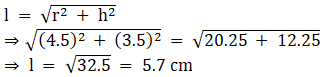The equation of volume of cone = 1/3πr2h

We know that,

Volume of rice = Volume of conical heap

Volume of rice = 1/3π(4.5)2(3.5)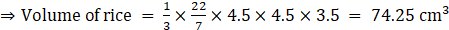We also know that,

Canvas requires to just cover heap = Curved surface area of conical heap

And curved surface area of a cone = πrl

Therefore, the canvas required = π(4.5)(5.7) = 80.61 cm2 [appx]

7. A factory manufactures 120000 pencils daily. The pencils are cylindrical in shape each of length 25 cm and circumference of base as 1.5 cm. Determine the cost of colouring the curved surfaces of the pencils manufactured in one day at Rs 0.05 per dm2.

Solution:

The shape of pencil = cylinder.

Let the radius of base = r cm

Circumference of base = 1.5 cm

Circumference of circle is 2πr = 1.5 cm

r = 1.5/2π cm

According to the question,

Height, h = 25 cm

We know that,

Curved surface area of cylinder = 2πrh

Curved surface area of pencil = 2π (1.5/2π) 25 = 37.5 cm2

1 cm = 0.1 dm

1 cm2 = 0.01 dm2

37.5 cm2 = 0.375 dm2

Cost for coloring 1 dm2 = Rs. 0.05

Cost for coloring 0.375 dm2 (i.e. 1 pencil) = Rs. 0.01875

Cost for coloring 120000 pencils = 120000 ×0.01875 = Rs. 2250

8. Water is flowing at the rate of 15 km/h through a pipe of diameter 14 cm into a cuboidal pond which is 50 m long and 44 m wide. In what time will the level of water in pond rise by 21 cm?

Solution: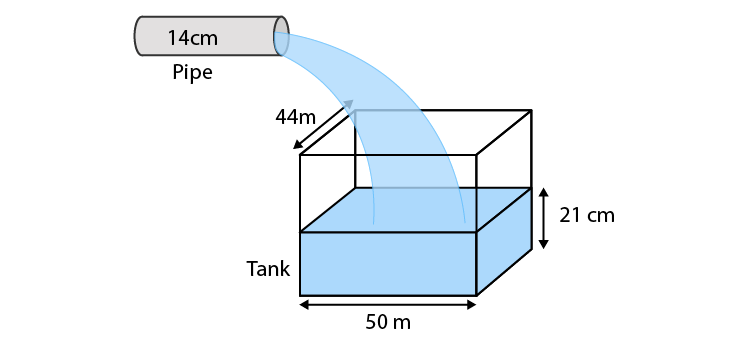Let the time taken by pipe to fill pond = t hours

Water flows 15 km in 1 hour, so, it will flow 15t meters in t hours.

We know that,

Volume of cuboidal pond up to height 21 cm = Volume of water that passes through pipe in “t” hours

Considering cuboidal pond,

Length, l = 50 m

Height, h = 21 cm = 0.21 m

We know that,

Volume of tank = lbh

Volume of water = 50(44)(0.21) = 462 m3

Considering cylindrical pipe

Base diameter = 14 cm

Base radius, r = 7 cm = 0.07 m

Height, h = 15t km = 15000t m

We also know that,

Volume of a cylinder = πr2h

Volume of water passed in pipe = π(0.07)2(15000t)

=22/7 × 0.07 × 0.07 × 15000t

= 231t cm3

So, we have

231t = 462

t = 2 hours

Time required to fill tank up to a height of 25 cm is 2 hours.

9. A solid iron cuboidal block of dimensions 4.4 m × 2.6 m × 1m is recast into a hollow cylindrical pipe of internal radius 30 cm and thickness 5 cm. Find the length of the pipe.

Solution:

Considering cuboidal block

Length, l = 4 m

Height, h = 1 m

We know that,

Volume of tank = lbh

Volume of cuboid = 4.4(2.6)(1) = 11.44 m3

We know that,

The volume remains same when a body is recast to another body.

According to the question,

Volume of cylindrical pipe = 11.44 m3

Considering pipe or the hollow cylinder

Internal radius, r2 = 30 cm = 0.3 m

Thickness = 5 cm

External radius, r1 = Internal radius + thickness = 30 + 5 = 35 cm = 0.35 m

Let the length of pipe = h

We know that,

Volume of a hollow cylinder = πh(r12 – r22)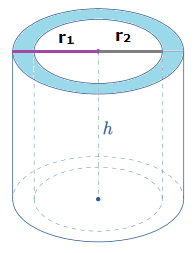Hence,

Volume of pipe = πh((0.35)2 – (0.3)2)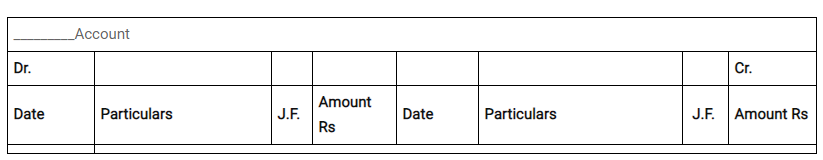So, the length of pipe is 112 m.

10. 500 persons are taking a dip into a cuboidal pond which is 80 m long and 50 m broad. What is the rise of water level in the pond, if the average displacement of the water by a person is 0.04m3?

Solution:

According to the question,

Average displacement by a person = 0.04 m3

Average displacement by 500 persons = 500 × 0.04 = 20 m3

Hence, the volume of water raised in pond = 20 m3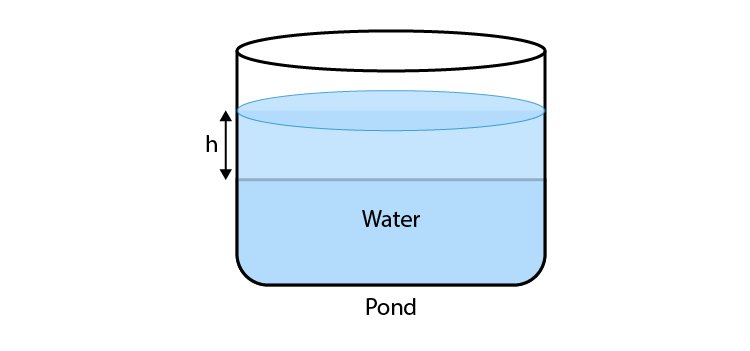It is also given that,

Length of pond, l = 80 m

Breadth of pond, b = 50 m

Height = h

Volume of water raised in pond = 80(50)(h)

20 m3 = 4000h

h = 0.005 m = 0.5 cm

Therefore, raise in the height of water = 0.5 cm.

Download BYJU’S – The Learning App to get personalised videos explaining different types of Maths topics such as surface areas and volumes of solid objects, etc., and experience a new approach to learning to understand the concepts in an easy way.

## Frequently Asked Questions on NCERT Exemplar Solutions for Class 10 Maths Chapter 12

Q1

### How many problems are there in each exercise of the NCERT Exemplar Solutions for Class 10 Maths Chapter 12?

Chapter 12 of the NCERT Solutions for Class 10 Maths has four exercises. Exercise 12.1 has 10 questions, Exercise 12.2 has 4 questions, and  Exercise 12.3 has 7 questions, whereas the last exercise has 10 questions. Each and every problem is solved with utmost care to provide students with accurate solutions as per the CBSE syllabus and guidelines.
Q2

### What do you mean by the frustum of a cone in Chapter 12 of NCERT Exemplar Solutions for Class 10 Maths?

The frustum of a cone is part of the cone when it is cut by a plane into two parts. The upper part of the cone remains the same in shape, but the bottom part makes a frustum. To get this part of the right circular cone, we have to slice it horizontally or parallel to the base. Both pieces have different volumes and areas.
Q3

### Where do I get NCERT Exemplar Solutions for Class 10 Maths Chapter 12 online?

You can download the NCERT Exemplar Solutions for Class 10 Maths Chapter 12 on BYJU’S website. The solutions to all the problems are curated by the subject experts to provide the students with the best reference material to rely on. You can download both chapter-wise or exercise-wise solutions and get a clear idea about the problem-solving techniques which are implemented while answering the textbook problems.